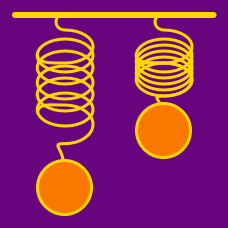Classical Mechanics

Periodic Motion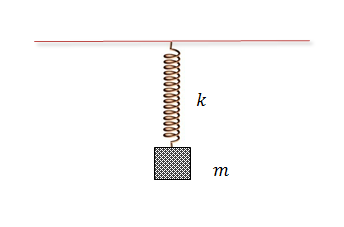A body of mass $m = 4 \text{ kg}$ is attached to a spring that hangs from the ceiling. If the spring is held at its original length and then let go, the body oscillates with amplitude $A = {\frac{a}{b}} \text{ m},$ where $a$ and $b$ are coprime positive integers. Find the value of $a+b.$

The spring constant is $k = 5 \text{ N/m}$ and the gravitational acceleration is $g=10\text{ m/s}^2.$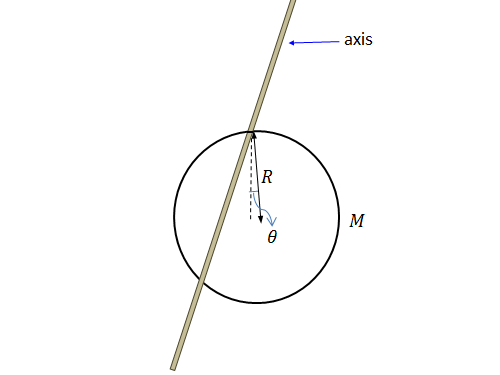A ring of mass $M = 5 \text{ kg}$ and radius $R = 3 \text{ m}$ oscillates on a horizontally placed rod. If the ring's angular frequency can be expressed as $\omega = \sqrt{\frac{a}{b}} \text{ rad/s},$ where $a$ and $b$ are coprime positive integers, what is the value of $a+b?$

The moment of inertia is given by $I = 2MR^2$ and the gravitational acceleration is $g=10\text{ m/s}^2.$A body of mass $m = 8 \text{ kg}$ oscillates on a spring hanging from the ceiling with an angular frequency of $\omega = \sqrt{\frac{a}{b}} \text{ rad/s},$ where $a$ and $b$ are coprime positive integers. Find the value of $a+b.$

The spring constant is $k = 5 \text{ N/m}$ and the gravitational acceleration is $g=10\text{ m/s}^2.$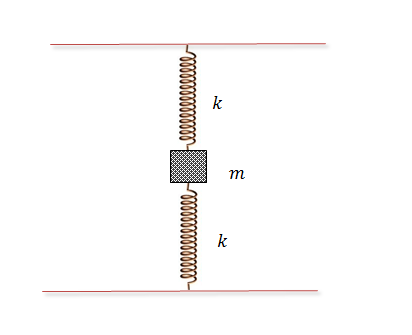In the figure above, a body of mass $m = 4 \text{ kg}$ oscillates in the middle of two identical springs with an angular speed of $\omega = \sqrt{\frac{a}{b}} \text{ rad/s},$ where $a$ and $b$ are coprime positive integers. Find the value of $a+b.$

The spring constant is $k = 9 \text{ N/m}$ and the gravitational acceleration is $g=10\text{ m/s}^2.$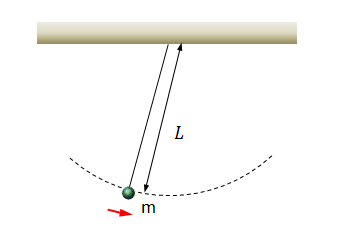A pendulum of mass $m = 5 \text{ kg}$ and length $L = 12 \text{ m}$ oscillates with an angular frequency of $\omega = \sqrt{\frac{a}{b}} \text{ rad/s},$ where $a$ and $b$ are coprime positive integers. Find the value of $a+b.$

The gravitational acceleration is $g=10\text{ m/s}^2.$

×